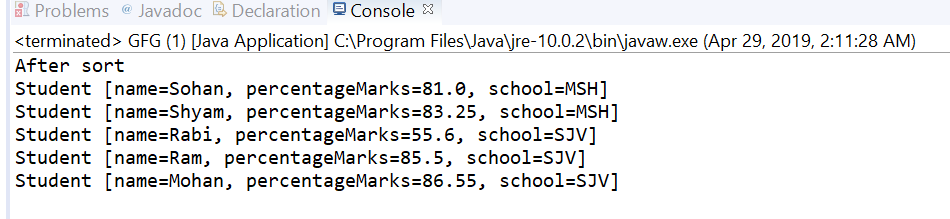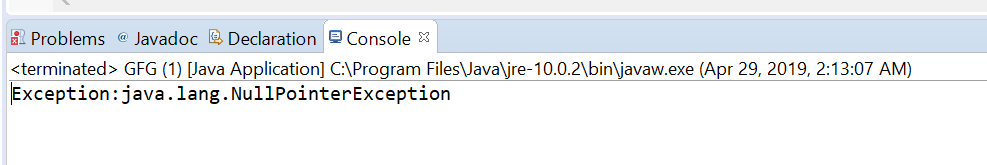Open In App

# Comparator thenComparingDouble() method in Java with examples

The thenComparingDouble(java.util.function.ToDoubleFunction) method of Comparator Interface in Java returns a lexicographic-order comparator with a function that extracts a double sort key. This method is applied after comparing method if you want to apply another comparing for those values which are equal in the comparing method.

Syntax:

```default Comparator <T> thenComparingDouble(
ToDoubleFunction <T> keyExtractor)
```

Parameters: This method accepts keyExtractor which is the function used to extract the Double sort key.

Return value: This method returns a lexicographic-order comparator composed of this and then the Double sort key.

Exception: This method throws NullPointerException if the argument is null.

Below programs illustrate thenComparingDouble(java.util.function.ToDoubleFunction) method:
Program 1:

 `// Java program to demonstrate Comparator``// thenComparingDouble(ToDoubleFunction) method`` ` `import` `java.util.Arrays;``import` `java.util.Collections;``import` `java.util.Comparator;``import` `java.util.List;`` ` `public` `class` `GFG {`` ` `    ``public` `static` `void` `main(String[] args)``    ``{``        ``List list = getStudentList();`` ` `        ``Comparator comparator``            ``= Comparator``                  ``.comparing(Student::getSchool)``                  ``.thenComparingDouble(Student::getpercentageMarks);`` ` `        ``Collections.sort(list, comparator);``        ``System.out.println(``"After sort"``);``        ``list.forEach(s -> System.out.println(s));``    ``}`` ` `    ``public` `static` `List getStudentList()``    ``{`` ` `        ``Student s1 = ``new` `Student(``"Ram"``, ``85.5``, ``"SJV"``);``        ``Student s2 = ``new` `Student(``"Shyam"``, ``83.25``, ``"MSH"``);``        ``Student s3 = ``new` `Student(``"Mohan"``, ``86.55``, ``"SJV"``);``        ``Student s4 = ``new` `Student(``"Sohan"``, ``81.00``, ``"MSH"``);``        ``Student s5 = ``new` `Student(``"Rabi"``, ``55.6``, ``"SJV"``);``        ``List list = Arrays.asList(s1, s2, s3, s4, s5);`` ` `        ``return` `list;``    ``}``}`` ` `class` `Student {`` ` `    ``private` `String name;``    ``private` `double` `percentageMarks;``    ``private` `String school;`` ` `    ``public` `Student(String name,``                   ``double` `percentageMarks,``                   ``String school)``    ``{``        ``this``.name = name;``        ``this``.percentageMarks = percentageMarks;``        ``this``.school = school;``    ``}`` ` `    ``public` `String getName()``    ``{``        ``return` `name;``    ``}`` ` `    ``public` `void` `setName(String name)``    ``{``        ``this``.name = name;``    ``}`` ` `    ``public` `double` `getpercentageMarks()``    ``{``        ``return` `percentageMarks;``    ``}`` ` `    ``public` `void` `setpercentageMarks(``int` `percentageMarks)``    ``{``        ``this``.percentageMarks = percentageMarks;``    ``}`` ` `    ``public` `String getSchool()``    ``{``        ``return` `school;``    ``}`` ` `    ``public` `void` `setSchool(String school)``    ``{``        ``this``.school = school;``    ``}`` ` `    ``@Override``    ``public` `String toString()``    ``{``        ``return` `"Student [name="` `+ name + ", ``            ``percentageMarks``            ``= " + percentageMarks ``              ``+ ``", school="` `+ school + ``"]"``;``    ``}``}`

The output printed on console of IDE is shown below.
Output:You can see in example first sorting is done on school wise and if the school is same then percentageMarks wise.

Program 2:

 `// Java program to demonstrate Comparator``// thenComparingDouble(ToDoubleFunction)  method`` ` `import` `java.util.Arrays;``import` `java.util.Comparator;``import` `java.util.List;`` ` `public` `class` `GFG {`` ` `    ``public` `static` `void` `main(String... args)``    ``{`` ` `        ``List list``            ``= Arrays.asList(``1``, ``2``, ``3``, ``4``, ``5``, ``6``);`` ` `        ``try` `{`` ` `            ``// apply thenComparingDouble``            ``Comparator.comparing(list::get)``                ``.thenComparingDouble(``null``);``        ``}``        ``catch` `(Exception e) {`` ` `            ``System.out.printf(``"Exception:"` `+ e);``        ``}``    ``}``}`

The output printed on console is shown below.
Output: